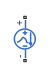# Exponential Current Source

Exponential pulse current source

•Libraries:
Simscape / Electrical / Additional Components / SPICE Sources

## Description

The Exponential Current Source block represents a current source whose output current value is an exponential pulse as a function of time and is independent of the voltage across the terminals of the source. The following equations describe the current through the source as a function of time:

`$\begin{array}{l}{I}_{out}\left(0\le Time\le TDR\right)\right)=I1\\ {I}_{out}\left(TDR`

where:

• I1 is the Initial value, I1 parameter value.

• I2 is the Pulse value, I2 parameter value.

• TDR is the Rise delay time, TDR parameter value.

• TR is the Rise time, TR parameter value.

• TDF is the Fall delay time, TDF parameter value.

• TF is the Fall time, TF parameter value.

The block uses a small conductance internally to prevent numerical simulation issues. The conductance connects the + and - ports of the device and has a conductance GMIN:

• By default, GMIN matches the GMIN parameter of the Environment Parameters block, whose default value is `1e–12`.

• To change GMIN, add an Environment Parameters block to your model and set the GMIN parameter to the desired value.

## Ports

### Conserving

expand all

Electrical conserving port associated with the DC current source positive voltage

Electrical conserving port associated with the DC current source negative voltage

## Parameters

expand all

Value of the output current at time zero, in A.

Asymptotic value of the output current when the output is high, in A.

Rise time delay, in s.

Time it takes the output current to rise from the Initial Value, I1 value to the Pulse Value, I2 value.

Fall time delay. The default value is `0``s`, which differs from the SPICE default value.

Time it takes the output current to fall from the Pulse value, I2 value to the Initial value, I1 value.

Specify if the current source includes a parallel conductance. The value of the parallel conductance, GMIN, depends on whether your model contains an Environment Parameters block.

This table shows the possible values of the parallel conductance, GMIN:

Values of parallel conductance, GMIN

Include parallel conductance, GMIN
`Yes``No`
Model contains Environment Parameters blockModel does not contain Environment Parameters blockParallel conductance, GMIN, is not included and it does not affect the current source.
GMIN is equal to the value of the GMIN parameter in the Environment Parameters block.GMIN = `1e-12` `Ohm`

## Version History

Introduced in R2008a